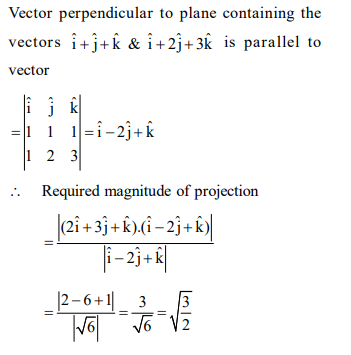# The magnitude of the projection of the vector`
Question:

The magnitude of the projection of the vector $2 \hat{\mathrm{i}}+3 \hat{\mathrm{j}}+\hat{\mathrm{k}}$ on the vector perpendicular to the plane containing the vectors $\hat{i}+\hat{j}+\hat{k}$ and $\hat{i}+2 \hat{j}+3 \hat{k}$, is:

1. $\frac{\sqrt{3}}{2}$

2. $\sqrt{\frac{3}{2}}$

3. $\sqrt{6}$

4. $3 \sqrt{6}$

Correct Option: , 2

Solution: